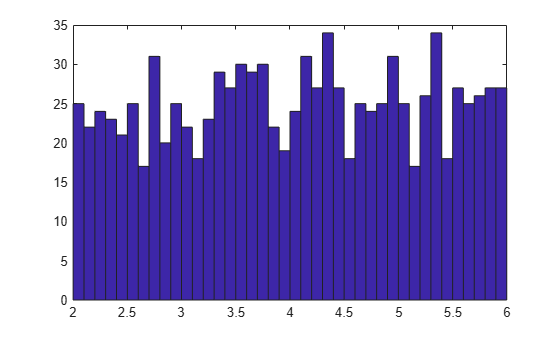## Uncertain Real Parameters

An uncertain real parameter, `ureal`, is the Control Design Block that represents a real number whose value is uncertain.

### Properties of Uncertain Real Parameters

Uncertain real parameters have a name (the `Name` property), and a nominal value (the `NominalValue` property). Several other properties (`PlusMinus`, `Range`, `Percentage`) describe the uncertainty in parameter values.

All properties of a `ureal` can be accessed through `get` and `set`. The properties are:

Properties

Meaning

Class

`Name`

Internal name

`char`

`NominalValue`

Nominal value of element

`double`

`Mode`

Signifies which description (from`'PlusMinus'`, `'Range'`, `'Percentage'`) of uncertainty is invariant when `NominalValue` is changed

`char`

`PlusMinus`

`scalar or 1x2` `double`

`Range`

Numerical range

`1x2` `double`

`Percentage`

Additive variation (% of absolute value of nominal)

`scalar or 1x2 double`

`AutoSimplify`

`'off'```` | {````'basic'````} |````'full'`

`char`

The properties `Range`, `Percentage` and `PlusMinus` are all automatically synchronized. If the nominal value is 0, then the `Mode` cannot be `Percentage`. The `Mode` property controls what aspect of the uncertainty remains unchanged when `NominalValue` is changed. Assigning to any of `Range`/`Percentage`/`PlusMinus` changes the value, but does not change the mode.

The `AutoSimplify` property controls how expressions involving the real parameter are simplified. Its default value is `'basic'`, which means elementary methods of simplification are applied as operations are completed. Other values for `AutoSimplify` are `'off'` (no simplification performed) and `'full'` (model-reduction-like techniques are applied). See Simplifying Representation of Uncertain Objects to learn more about the `AutoSimplify` property and the command `simplify`.

If no property/value pairs are specified, default values are used. The default `Mode` is `PlusMinus`, and the default value of `PlusMinus` is `[-1 1]`. Some examples are shown below. In many cases, the full property name is not specified, taking advantage of the case-insensitive, partial name property matching.

### Create Uncertain Real Parameters

This example shows how to create uncertain real parameters, modify properties such as range of uncertainty, and sample uncertain parameters.

Create an uncertain real parameter, nominal value 3, with default values for all unspecified properties (including plus/minus variability of 1).

`a = ureal('a',3)`
```Uncertain real parameter "a" with nominal value 3 and variability [-1,1]. ```

View the properties and their values, and note that the `Range` and `Percentage` descriptions of variability are automatically maintained.

`get(a)`
``` NominalValue: 3 Mode: 'PlusMinus' Range: [2 4] PlusMinus: [-1 1] Percentage: [-33.3333 33.3333] AutoSimplify: 'basic' Name: 'a' ```

Create an uncertain real parameter, nominal value 2, with 20% variability. Again, view the properties, and note that the `Range` and `PlusMinus` descriptions of variability are automatically maintained.

`b = ureal('b',2,'Percentage',20) `
```Uncertain real parameter "b" with nominal value 2 and variability [-20,20]%. ```
`get(b)`
``` NominalValue: 2 Mode: 'Percentage' Range: [1.6000 2.4000] PlusMinus: [-0.4000 0.4000] Percentage: [-20 20] AutoSimplify: 'basic' Name: 'b' ```

Change the range of the parameter. All descriptions of variability are automatically updated, while the nominal value remains fixed. Although the change in variability was accomplished by specifying the `Range`, the `Mode` is unaffected, and remains `Percentage`.

```b.Range = [1.9 2.3]; get(b)```
``` NominalValue: 2 Mode: 'Percentage' Range: [1.9000 2.3000] PlusMinus: [-0.1000 0.3000] Percentage: [-5.0000 15.0000] AutoSimplify: 'basic' Name: 'b' ```

As mentioned, the `Mode` property signifies what aspect of the uncertainty remains unchanged when `NominalValue` is modified. Hence, if a real parameter is in `Percentage` mode, then the `Range` and `PlusMinus` properties are determined from the `Percentage` property and `NominalValue`. Changing `NominalValue` preserves the `Percentage` property, and automatically updates the `Range` and `PlusMinus` properties.

```b.NominalValue = 2.2; get(b)```
``` NominalValue: 2.2000 Mode: 'Percentage' Range: [2.0900 2.5300] PlusMinus: [-0.1100 0.3300] Percentage: [-5.0000 15.0000] AutoSimplify: 'basic' Name: 'b' ```

Create an uncertain parameter with an asymmetric variation about its nominal value. Examine the properties to confirm the asymmetric range.

```c = ureal('c',-5,'Percentage',[-20 30]); get(c)```
``` NominalValue: -5 Mode: 'Percentage' Range: [-6 -3.5000] PlusMinus: [-1 1.5000] Percentage: [-20 30] AutoSimplify: 'basic' Name: 'c' ```

Create an uncertain parameter, specifying variability with `Percentage`, but force the `Mode` to be `Range`.

```d = ureal('d',-1,'Mode','Range','Percentage',[-40 60]); get(d)```
``` NominalValue: -1 Mode: 'Range' Range: [-1.4000 -0.4000] PlusMinus: [-0.4000 0.6000] Percentage: [-40 60] AutoSimplify: 'basic' Name: 'd' ```

Finally, create an uncertain real parameter, and set the `AutoSimplify` property to `'full'`.

`e = ureal('e',10,'PlusMinus',[-23],'Mode','Percentage','AutoSimplify','Full') `
```Uncertain real parameter "e" with nominal value 10 and variability [-230,230]%. ```
`get(e)`
``` NominalValue: 10 Mode: 'Percentage' Range: [-13 33] PlusMinus: [-23 23] Percentage: [-230 230] AutoSimplify: 'full' Name: 'e' ```

Specifying conflicting values for `Range/Percentage/PlusMinus` when creating a `ureal` element does not result in an error. In this case, the last specified property is used. This last occurrence also determines the `Mode`, unless `Mode` is explicitly specified, in which case that is used, regardless of the property/value pairs ordering.

`f = ureal('f',3,'PlusMinus',[-2 1],'Percentage',40) `
```Uncertain real parameter "f" with nominal value 3 and variability [-40,40]%. ```
`g = ureal('g',2,'PlusMinus',[-2 1],'Mode','Range','Percentage',40) `
```Uncertain real parameter "g" with nominal value 2 and range [1.2,2.8]. ```
`g.Mode`
```ans = 'Range' ```

Create an uncertain real parameter, use `usample` to generate 1000 instances (resulting in a 1-by-1-by-1000 array), reshape the array, and plot a histogram, with 20 bins (within the range of 2 to 4).

```h = ureal('h',3); hsample = usample(h,1000); hist(reshape(hsample,[1000 1]),20);```Make the range nonsymmetric about the nominal value, and repeat the sampling, and histogram plot (with 40 bins over the range of 2-to-6)

```h.Range = [2 6]; hsample = usample(h,1000); hist(reshape(hsample,[1000 1]),40);```The distribution is still uniform. The distribution used by `usample` is uniform in the actual value of the uncertain real parameter. However, highly skewed ranges can lead to poor numeric conditioning and poor results. Therefore, for meaningful results, avoid highly skewed ranges where the nominal value is orders of magnitude closer to one end of the range than to the other.

There is no notion of an empty `ureal` (or any other uncertain element, for that matter). `ureal`, by itself, creates an element named `'UNNAMED'`, with default property values.